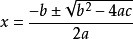# 如何实现高抛或平抛发射？• 起点发射速度大小 `V`
• 重力加速 `G`
• 起始点与经过点

• 发射角度 `a```````const s = location.x - START_POS.x;
const h = location.y - START_POS.y;
// a*t^2 + b*t + c = 0
const a = G * s / (2 * V * V);
const b = 1;
const c = a - h / s;
const delta = b * b - 4 * a * c;
if (delta >= 0) {
// 一元二次方程求根公式
const t1 = (-b + Math.sqrt(delta)) / (2 * a); // 平抛 tan 值
const t2 = (-b - Math.sqrt(delta)) / (2 * a); // 高抛 tan 值
// 二、四象限角度要加 180
const alpha1 = Math.atan(t1) + (s < 0 ? Math.PI : 0);
const alpha2 = Math.atan(t2) + (s < 0 ? Math.PI : 0);
}
``````

``````const v_x_1 = Math.cos(alpha1) * V;
const v_y_1 = Math.sin(alpha1) * V;
const v_x_2 = Math.cos(alpha2) * V;
const v_y_2 = Math.sin(alpha2) * V;
// 低抛线速度 保存起来
this._linearVelocity_1.x = v_x_1;
this._linearVelocity_1.y = v_y_1;
// 高抛线速度 保存起来
this._linearVelocity_2.x = v_x_2;
this._linearVelocity_2.y = v_y_2;
``````

``````// 计算夹角
const angle = rigidBody.linearVelocity.clone().signAngle(cc.v2(1, 0));
rigidBody.node.rotation = angle * 180 / Math.PI;
``````

``````this.schedule(this.fireArrow, 0.5, cc.macro.REPEAT_FOREVER);
``````

``````private _index = 0;
private fireArrow() {
const linearVelocity = this.toggle_arrow.isChecked ? this._linearVelocity_2.clone() : this._linearVelocity_1.clone()
const rigidBody_arrow = this._all_arrows[this._index++ % this._all_arrows.length];
rigidBody_arrow.node.setPosition(START_POS);
rigidBody_arrow.linearVelocity = linearVelocity;
}
``````

29赞

mark

mark

mark

mark

mark## 抛物线的瞄准线的绘制！

mark一下

mark

make

make 一下3d 的就分3个方向去计算，都是匀加速直线运动。